Differential Calculus NAT Level - 1

# Differential Calculus NAT Level - 1

Test Description

## 10 Questions MCQ Test Topic wise Tests for IIT JAM Physics | Differential Calculus NAT Level - 1

Differential Calculus NAT Level - 1 for IIT JAM 2023 is part of Topic wise Tests for IIT JAM Physics preparation. The Differential Calculus NAT Level - 1 questions and answers have been prepared according to the IIT JAM exam syllabus.The Differential Calculus NAT Level - 1 MCQs are made for IIT JAM 2023 Exam. Find important definitions, questions, notes, meanings, examples, exercises, MCQs and online tests for Differential Calculus NAT Level - 1 below.
Solutions of Differential Calculus NAT Level - 1 questions in English are available as part of our Topic wise Tests for IIT JAM Physics for IIT JAM & Differential Calculus NAT Level - 1 solutions in Hindi for Topic wise Tests for IIT JAM Physics course. Download more important topics, notes, lectures and mock test series for IIT JAM Exam by signing up for free. Attempt Differential Calculus NAT Level - 1 | 10 questions in 30 minutes | Mock test for IIT JAM preparation | Free important questions MCQ to study Topic wise Tests for IIT JAM Physics for IIT JAM Exam | Download free PDF with solutions
 1 Crore+ students have signed up on EduRev. Have you?
*Answer can only contain numeric values
Differential Calculus NAT Level - 1 - Question 1

### If f"(x) > 0 and f'(1) = 0 such that g(x) = f(cot2 x + 2cot x + 2) where 0 < x < π, then g(x) decreasing in (a, b) where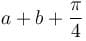is

Detailed Solution for Differential Calculus NAT Level - 1 - Question 1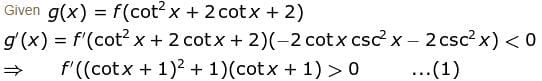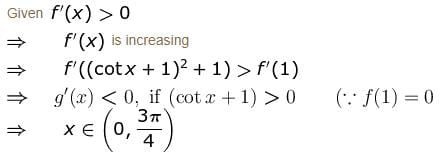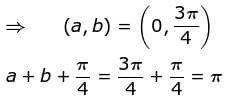*Answer can only contain numeric values
Differential Calculus NAT Level - 1 - Question 2

### If f(x) has a maximum or a minimum at a point x0 inside the interval, then f '(x0) equals :

Detailed Solution for Differential Calculus NAT Level - 1 - Question 2

It is necessary that at the point of maxima or minima of a function, say ff ' will become zero.

*Answer can only contain numeric values
Differential Calculus NAT Level - 1 - Question 3

### If 1" = α radians, then the approximate value of cos 60°1' is given as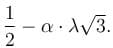Find the value of  λ.

Detailed Solution for Differential Calculus NAT Level - 1 - Question 3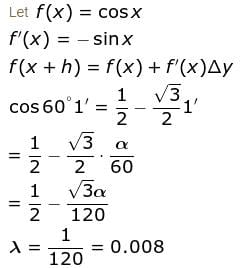*Answer can only contain numeric values
Differential Calculus NAT Level - 1 - Question 4

The maximum value of u is, where u = axy2z2 - x2y2z3 - xy3z3 - xy2z4 is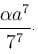Find the value of α.

Detailed Solution for Differential Calculus NAT Level - 1 - Question 4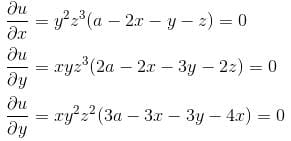Solving, we get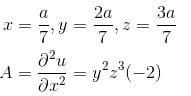Thus, max value is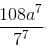*Answer can only contain numeric values
Differential Calculus NAT Level - 1 - Question 5

The sum of one number and three times a second number is 60. Find the pair, where product is maximum.

Detailed Solution for Differential Calculus NAT Level - 1 - Question 5

Let the two numbers be x & y

⇒ x + 3y = 60 ....(1)

Now, let z = xy = product of two numbers.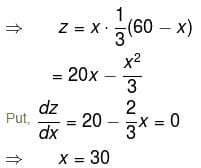Putting x = 30 in (1), we get y = 10 and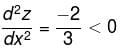Hence, z is maximum when x = 30 and y = 10.

*Answer can only contain numeric values
Differential Calculus NAT Level - 1 - Question 6

Let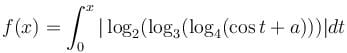be increasing for all real values of x, then range of a is  (α, ∞). Find value of α.

Detailed Solution for Differential Calculus NAT Level - 1 - Question 6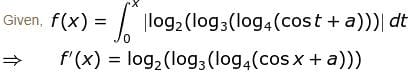Since f(x) is a function so,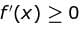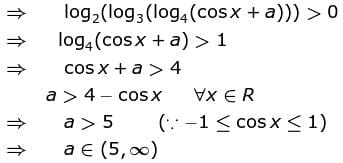Value of α = 5

*Answer can only contain numeric values
Differential Calculus NAT Level - 1 - Question 7

The greatest and the least value of the function f(x) = x3 – 18x2 + 96x in the interval [0, 9] are :

Detailed Solution for Differential Calculus NAT Level - 1 - Question 7

f(x) = x3 – 18x2 + 96x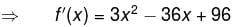= 3(x – 4)(x – 8)
Put f'(x) = 0, we get x = 4, 8
f''(x) = 6x – 36 = 6(x – 6)
which is positive at x = 8 and negative at x = 4

Now, f(0) = 0,  f(8) = 128
f(4) = 160,      f(9) = 135
∴  least value is 0 and greatest value is 160.

*Answer can only contain numeric values
Differential Calculus NAT Level - 1 - Question 8

Let f(x, y) = x4 + y4 - 2x2 + 4xy - 2y2 has a minimum at (-√α, √α) and (√α, - √α) Find the value of α.

Detailed Solution for Differential Calculus NAT Level - 1 - Question 8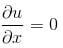⇒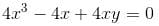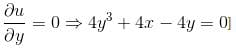Solving, we get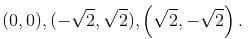r = 12x2 - 4
s = 4
t = 12y2 – 4
at (-√2, √2)
r = 20, s = 4, t = 20
rt - s2 > 0 and r > 0  ∴ minimum

*Answer can only contain numeric values
Differential Calculus NAT Level - 1 - Question 9

If the function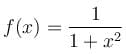is downward concave is (α, β) the [β - α] is

Detailed Solution for Differential Calculus NAT Level - 1 - Question 9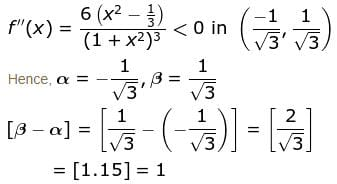∵  [.] greatest integer function

*Answer can only contain numeric values
Differential Calculus NAT Level - 1 - Question 10

The minimum value of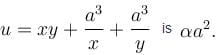Find the value of α.

Detailed Solution for Differential Calculus NAT Level - 1 - Question 10

For max. or min value of u,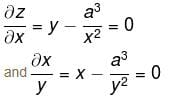Solving these equations, we get
x = y = a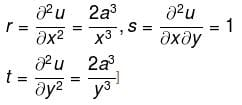at x = ay = a
r = 2, s = 1, t = 2
rt – s2 = 4 – 1 = 3 > 0 and r > 0

Therefore, u is min at x = ay = a and min. value is 3a2.

## Topic wise Tests for IIT JAM Physics

217 tests
Information about Differential Calculus NAT Level - 1 Page
In this test you can find the Exam questions for Differential Calculus NAT Level - 1 solved & explained in the simplest way possible. Besides giving Questions and answers for Differential Calculus NAT Level - 1, EduRev gives you an ample number of Online tests for practice

## Topic wise Tests for IIT JAM Physics

217 tests(Scan QR code)